# Assigning Repositories

By this part we are going to assign nine (9) groups of project repositories including one (1) group of the central. All of them will be managed by an instance to represesent the pattern of true-prime-pairs via bilateral 9 sums.The purpose of this instance is simulating the central behaviour on each pairs of human chromosomes. So the nine (9) groups would consist of three (3) containers + one (1) sub central + one (1) central + one (1) sub central + three (3) containers.

(7-1) + (12x9) = 6 + 108 = 114

``````  Tabulate Prime by Power of 10
loop(10) = π(10)-π(1) = 4-0 = 4
loop(100) = π(100)-π(10)-1th = 25-4-2 = 19
loop(1000) = π(1000) - π(100) - 10th = 168-25-29 = 114

-----------------------+----+----+----+----+----+----+----+----+----+-----
True Prime Pairs Δ    |  1 |  2 |  3 |  4 |  5 |  6 |  7 |  8 |  9 | Sum
=======================+====+====+====+====+====+====+====+====+====+=====
19 → π(10)            |  2 |  3 |  5 |  7 |  - |  - |  - |  - |  - | 4th  4 x Root
-----------------------+----+----+----+----+----+----+----+----+----+-----
17 → π(20)            | 11 | 13 | 17 | 19 |  - |  - |  - |  - |  - | 8th  4 x Twin
-----------------------+----+----+----+----+----+----+----+----+----+-----
13 → π(30) → 12 (Δ1)  | 23 | 29 |  - |  - |  - |  - |  - |  - |  - |10th
=======================+====+====+====+====+====+====+====+====+====+===== 1st Twin
11 → π(42)            | 31 | 37 | 41 |  - |  - |  - |  - |  - |  - |13th
-----------------------+----+----+----+----+----+----+----+----+----+----- 2nd Twin
7 → π(60) → 19 (Δ12) | 43 | 47 | 53 | 59 |  - |  - |  - |  - |  - |17th
-----------------------+----+----+----+----+----+----+----+----+----+----- 3rd Twin
5 → π(72) → 18 (Δ13) | 61 | 67 | 71 |  - |  - |  - |  - |  - |  - |20th
=======================+====+====+====+====+====+====+====+====+====+===== 4th Twin
3,2 → 18+13+12 → 43  | 73 | 79 | 83 | 89 | 97 | 101| 103| 107| 109|29th
=======================+====+====+====+====+====+====+====+====+====+=====
Δ                                                            Δ
12+13+(18+18)+13+12   ← 36th-Δ1=151-1=150=100+2x(13+12)   ←   30th = 113 = 114-1
``````

Let's sum them up so we get 9+1+9=19. Put them as one (1) unit so the whole objects will be 19 (sub objects)+1 (unit object)=20 objects. Thus this sum is what we called as bilateral 9 sums.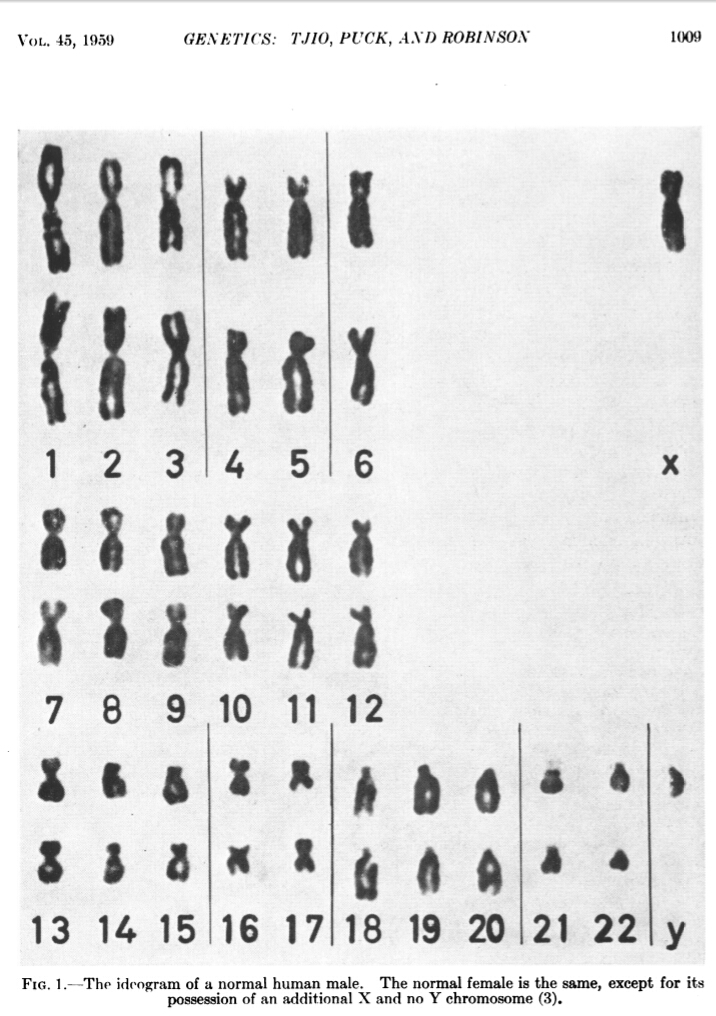As a matter of the fact every schemes are contained in X/Y genes where the Y gene has has hardly any genes. When we do pair them as reproduction genes then we will come again to 3 containers + 1 sub central + 1 central + 1 sub central + 3 containers.

The X bears about 1,600 genes with varied functions. But the Y has hardly any genes; maybe 50, and only 27 of these are in the male-specific part of the Y.(The Conversation)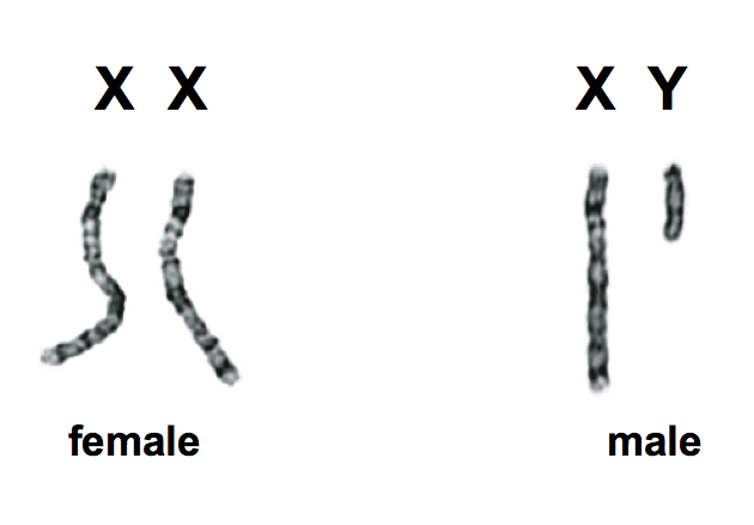``````                             --------------------------------------------------------------
|                                                              |
=======================+====+====+====+====+====+====+====+====+====+=====               |
19 → π(10)            |  2 |  3 |  5 |  7 |  - |  - |  - |  - |  - | 4th  4 x Root      |
-----------------------+----+----+----+----+----+----+----+----+----+-----               |
17 → π(20)            | 11 | 13 | 17 | 19 |  - |  - |  - |  - |  - | 8th  4 x Twin      |
-----------------------+----+----+----+----+----+----+----+----+----+-----               |
13 → π(30) → 12 (Δ1)  | 23 | 29 |  - |  - |  - |  - |  - |  - |  - |10th                |
=======================+====+====+====+====+====+====+====+====+====+===== 1st Twin      |
11 → π(42)            | 31 | 37 | 41 |  - |  - |  - |  - |  - |  - |13th                |
-----------------------+----+----+----+----+----+----+----+----+----+----- 2nd Twin      |
7 → π(60) → 19 (Δ12) | 43 | 47 | 53 | 59 |  - |  - |  - |  - |  - |17th                |
-----------------------+----+----+----+----+----+----+----+----+----+----- 3rd Twin      |
5 → π(72) → 18 (Δ13) | 61 | 67 | 71 |  - |  - |  - |  - |  - |  - |20th ---------------
=======================+====+====+====+====+====+====+====+====+====+===== 4th Twin
3,2 → 18+13+12 → 43  | 73 | 79 | 83 | 89 | 97 | 101| 103| 107| 109|29th
=======================+====+====+====+====+====+====+====+====+====+=====
``````

When recombination is occur then the prime 13 is forced to → 12 where the impact (Δ1) goes to 18+13+12=43 on the last 7th row. This sequence is simulated by a flowchart having 12 arrows flowing on 10 (ten) shapes of prime 31 up to 71 (40 nodes).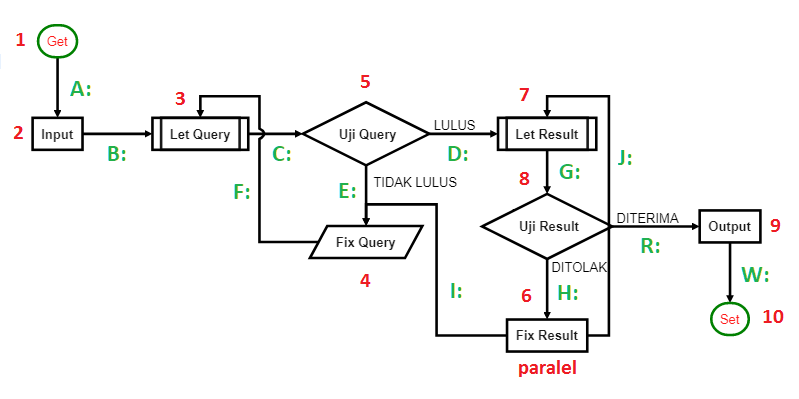This central doesn't exist phisically. However when we were going up by the form of nine (9) subs central + one (1) central + nine (9) subs central then we will get the human brain as the central.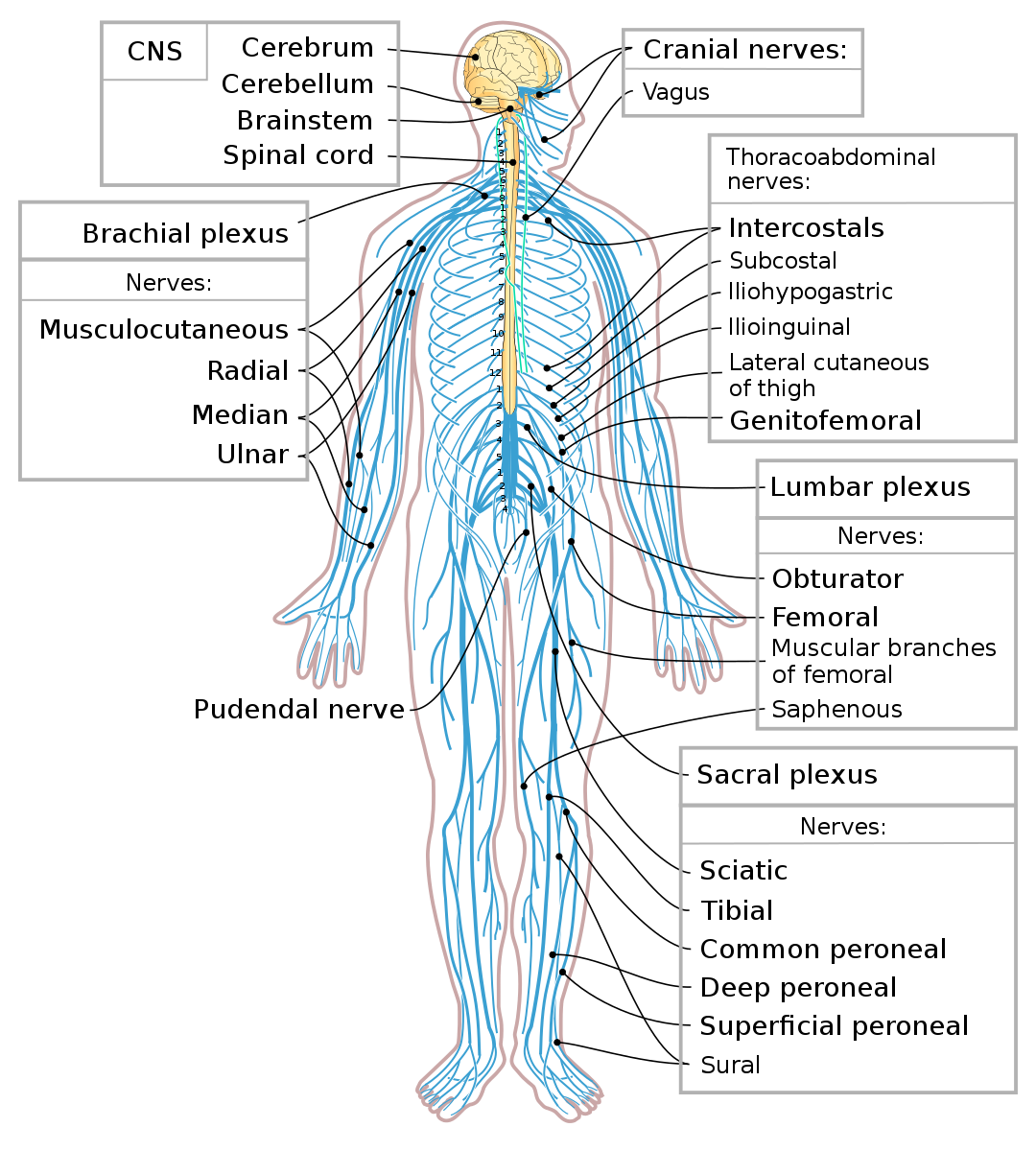Observing many literatures we finaly found that the pattern of this centralizing is done by a kind of neurotransmitter that works as a messenger by transmitting a signal. That is the way they are communicating each others.

A neurotransmitter is a signaling molecule secreted by a neuron to affect another cell across a synapse. The cell receiving the signal, any main body part or target cell, may be another neuron, but could also be a gland or muscle cell.. Wikipedia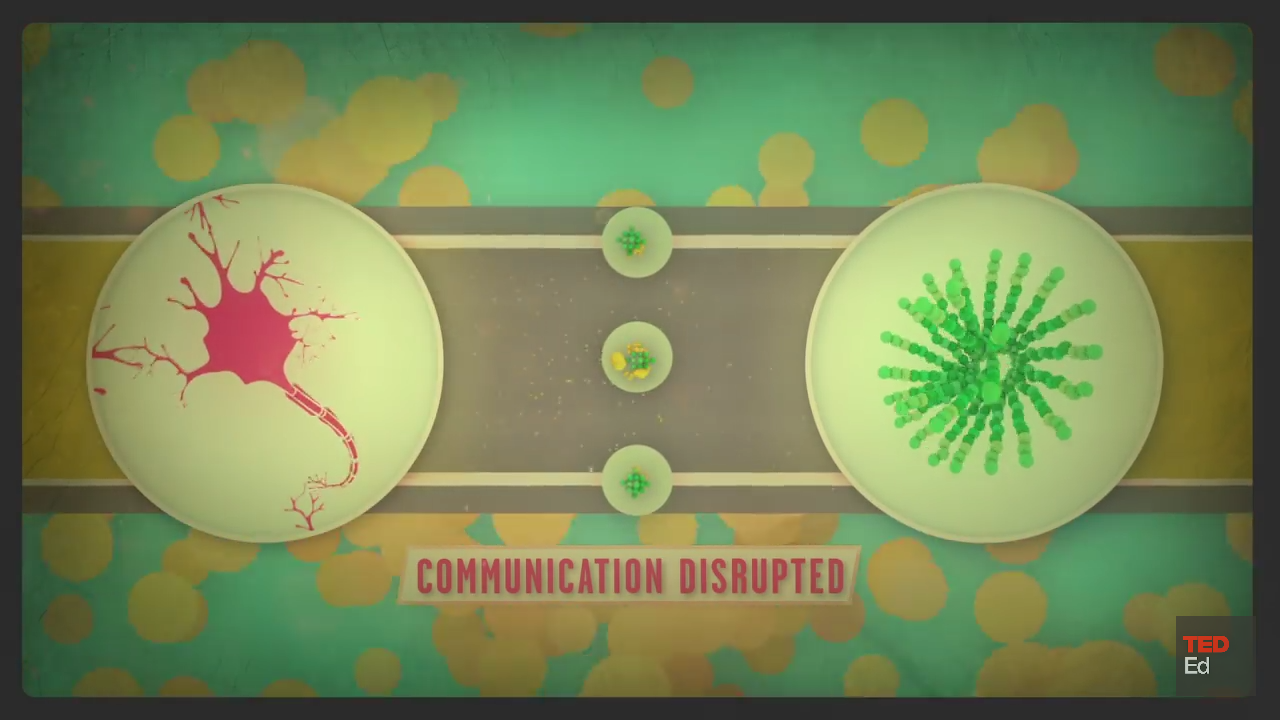However it seems that determining the pattern of this signalling either going up to the top of the brain or going down to the XX or XY genes even to the bottom of that little Y is staggeringly complex. Yet science points to a much more ambiguous reality.

Determination of biological sex is staggeringly complex, involving not only anatomy but an intricate choreography of genetic and chemical factors that unfolds over time. (ScientificAmerica)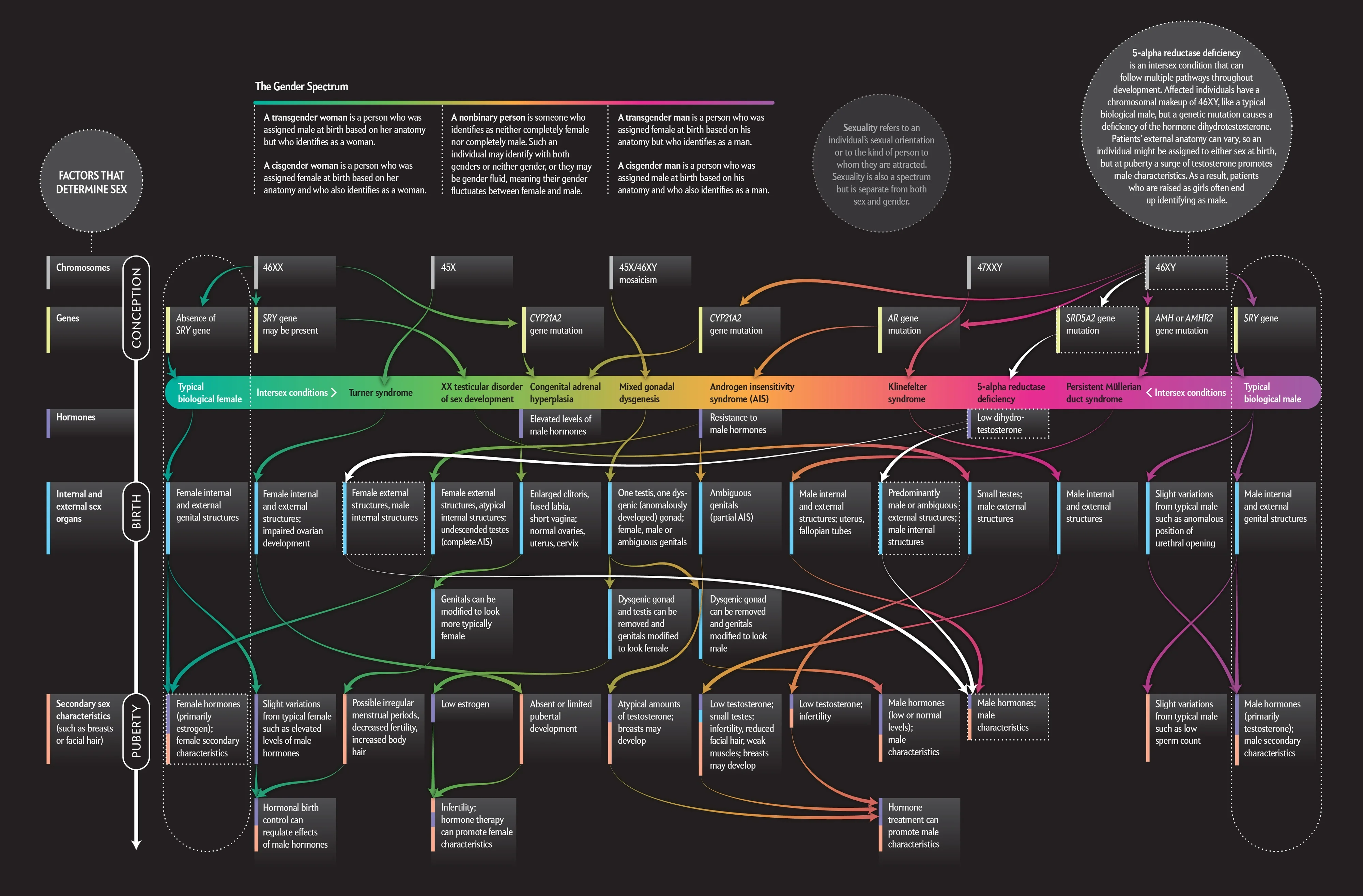Therefore here we would only take the fact that X bears about 1,600 genes with varied functions while the Y has 50, and only 27 of these are in the male-specific and how their relation with the 20 objects of bilateral 9 sum.